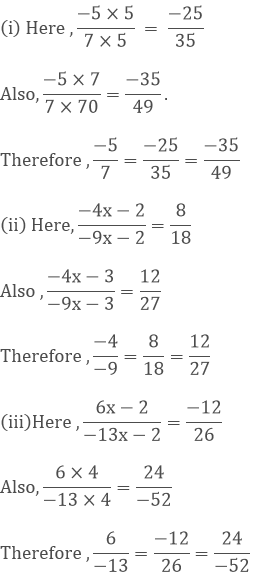×#### Thank you for registering.

One of our academic counsellors will contact you within 1 working day.

Click to Chat

1800-1023-196

+91-120-4616500

CART 0

• 0

MY CART (5)

Use Coupon: CART20 and get 20% off on all online Study Material

ITEM
DETAILS
MRP
DISCOUNT
FINAL PRICE
Total Price: Rs.

There are no items in this cart.
Continue Shopping• Complete JEE Main/Advanced Course and Test Series
• OFFERED PRICE: Rs. 15,900
• View Details

```Chapter 4: Rational Numbers Exercise – 4.3

Question: 1

Determine whether the following rational numbers are in the lowest form or not:

(i)  65/84

(ii)  (-15)/32

(iii) 24/128

(iv) (-56)/(-32)

Solution:

(i) We observe that 65 and 84 have no common factor their HCF is 1.

Thus, 65/84 is in its lowest form.

(ii) We observe that -15 and 32 have no common factor i.e., their HCF is 1.

Thus, -15/32 is in its lowest form.

(iii) HCF of 24 and 128 is not 1.

Thus, given rational number is not in its simplest form.

(iv) HCF of 56 and 32 is 8.

Thus, given rational number is not in its simplest form.

Question: 2

Express each of the following numbers to the lowest form:

(i)  4/22

(ii)  (- 36)/180

(iii)  132/428

(iv) 32/56

Solution:

Lowest form of:

(i)  4/22 is:

4 = 2 × 2, 22 = 2 × 11

HCF of 4 and 22 is 2.

Dividing the fraction by 2, we get 2/11.

(ii) -36/180 is:

36 = 3 × 3 × 2 × 2, 180 = 5 × 3 × 3 × 2 × 2

HCF of 36 and 180 is 36.

Dividing the fraction by 36, we get -1/5.

(iii) 132/- 428 is:

132 = 2 × 3 × 2 × 11, 428 = 2 × 2 × 107

HCF of 132 and 428 is 4.

Dividing the fraction by 4, we get 33/-107.

(iv) – 32/ – 56 is:

32 =2 × 2 × 2 × 2 × 2, 56 = 2 × 2 × 2 × 7

HCF of 32 and 56 is 8.

Dividing the fraction by 8, we get 4/7.

Question: 3

Fill in the blanks:Solution:```### Course Features

• 728 Video Lectures
• Revision Notes
• Previous Year Papers
• Mind Map
• Study Planner
• NCERT Solutions
• Discussion Forum
• Test paper with Video Solution